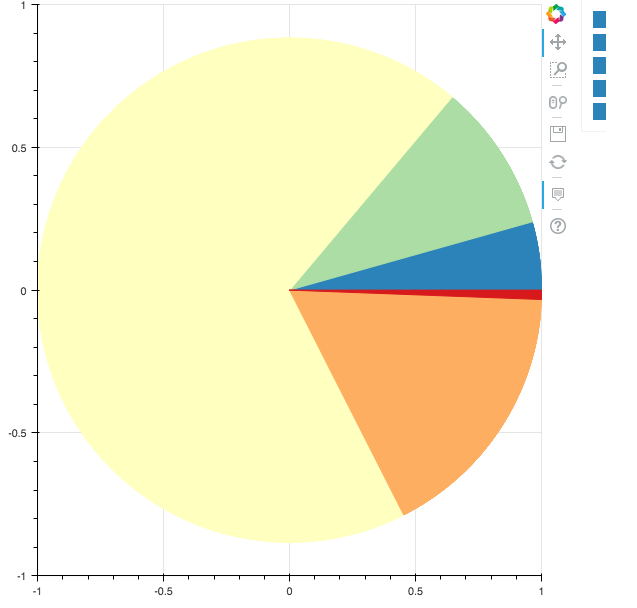# Pie chart with hover tooltips and legend outside of the plot area

Hello everyone!

I’m trying to do what the subject describes: a pie chart with hover tooltips and legend outside of the plot area.

I’ve walked a long distance following the examples on the Bokeh website, Stack Overflow and some threads over here

I’m almost there but I can’t make the colors on the legend to match the colors on the pie chart. Can anyone have a look at my code and suggest me what I need to change?

Note1: The legend labels don’t appear in this example because of some problem with the plot width but I know they are correct because in my application using Bokeh server they show up

Note2: The hover tooltip is fixed in the center of the pie. I’d like it to follow the mouse but I can bear with that after so much strugglingHere is the code and a screenshot of the resulting plot

from bokeh.io import curdoc

from bokeh.layouts import layout

from bokeh.models import (

HoverTool, ColumnDataSource, Legend, LegendItem

)

from bokeh.plotting import figure, show

from bokeh.palettes import brewer

from numpy import pi

starts = [0.0, 0.27429672626751683, 0.8713156234544629, 5.1821330567582686, 6.245873609350836]

ends = [0.27429672626751683, 0.8713156234544629, 5.1821330567582686, 6.245873609350836, 6.2831853071795862]

labels = [‘a’, ‘b’, ‘c’, ‘d’, ‘e’]

colors = [’#2b83ba’, ‘#abdda4’, ‘#ffffbf’, ‘#fdae61’, ‘#d7191c’]

amounts = [‘1,521,377’, ‘3,311,344’, ‘23,909,795’, ‘5,899,999’, ‘206,948’]

source=ColumnDataSource(dict(starts=starts, ends=ends, labels=labels, colors=colors, amounts=amounts))

plot = figure()

hover = HoverTool(

``````    tooltips=[

('type', '@labels'),

('quantity','@amounts')

]

)
``````

portions =

for i in range(len(starts)):

portions.append(plot.wedge(x=0, y=0, radius=1, start_angle=‘starts’, end_angle=‘ends’, color=‘colors’, source=source))

legend_items =

for idx, portion in enumerate(portions):

legend_items.append(LegendItem(label=labels[idx], renderers=[portion]))

legend = Legend(items=legend_items, location=(40, 0))

layout = layout([

``````[plot],
``````

])

show(plot)Hi,

For this you should be able to use the "auto-grouped" legends, which is fairly new and not as well documented as it could be (there are still some kinks to work out). But here is a version that should do what you are looking for:

from bokeh.io import output_file, show
from bokeh.layouts import layout
from bokeh.models import (
HoverTool, ColumnDataSource, Legend, LegendItem
)
from bokeh.plotting import figure
from bokeh.palettes import brewer
from numpy import pi

starts = [0.0, 0.27429672626751683, 0.8713156234544629, 5.1821330567582686, 6.245873609350836]
ends = [0.27429672626751683, 0.8713156234544629, 5.1821330567582686, 6.245873609350836, 6.2831853071795862]
labels = ['a', 'b', 'c', 'd', 'e']
colors = ['#2b83ba', '#abdda4', '#ffffbf', '#fdae61', '#d7191c']
amounts = ['1,521,377', '3,311,344', '23,909,795', '5,899,999', '206,948']

source=ColumnDataSource(dict(starts=starts, ends=ends, labels=labels, colors=colors, amounts=amounts))

plot = figure(toolbar_location="above")

hover = HoverTool(
tooltips=[
('type', '@labels'),
('quantity','@amounts')
]
)

r = plot.wedge(0, 0, radius=1, start_angle='starts', end_angle='ends', color='colors', source=source)

legend = Legend(items=[LegendItem(label=dict(field="labels"), renderers=[r])], location=(0, 0))

layout = layout([[plot]])

output_file("foo.html")

show(plot)

···

On Jun 8, 2017, at 05:22, [email protected] wrote:

Hello everyone!

I'm trying to do what the subject describes: a pie chart with hover tooltips and legend outside of the plot area.

I've walked a long distance following the examples on the Bokeh website, Stack Overflow and some threads over here

I'm almost there but I can't make the colors on the legend to match the colors on the pie chart. Can anyone have a look at my code and suggest me what I need to change?

Note1: The legend labels don't appear in this example because of some problem with the plot width but I know they are correct because in my application using Bokeh server they show up
Note2: The hover tooltip is fixed in the center of the pie. I'd like it to follow the mouse but I can bear with that after so much strugglingHere is the code and a screenshot of the resulting plot

from bokeh.io import curdoc
from bokeh.layouts import layout
from bokeh.models import (
HoverTool, ColumnDataSource, Legend, LegendItem
)
from bokeh.plotting import figure, show
from bokeh.palettes import brewer
from numpy import pi

starts = [0.0, 0.27429672626751683, 0.8713156234544629, 5.1821330567582686, 6.245873609350836]
ends = [0.27429672626751683, 0.8713156234544629, 5.1821330567582686, 6.245873609350836, 6.2831853071795862]
labels = ['a', 'b', 'c', 'd', 'e']
colors = ['#2b83ba', '#abdda4', '#ffffbf', '#fdae61', '#d7191c']
amounts = ['1,521,377', '3,311,344', '23,909,795', '5,899,999', '206,948']

source=ColumnDataSource(dict(starts=starts, ends=ends, labels=labels, colors=colors, amounts=amounts))

plot = figure()

hover = HoverTool(
tooltips=[
('type', '@labels'),
('quantity','@amounts')
]
)

portions =
for i in range(len(starts)):
portions.append(plot.wedge(x=0, y=0, radius=1, start_angle='starts', end_angle='ends', color='colors', source=source))

legend_items =
for idx, portion in enumerate(portions):
legend_items.append(LegendItem(label=labels[idx], renderers=[portion]))

legend = Legend(items=legend_items, location=(40, 0))

layout = layout([
[plot],
])

show(plot)

--
You received this message because you are subscribed to the Google Groups "Bokeh Discussion - Public" group.
To unsubscribe from this group and stop receiving emails from it, send an email to [email protected].
To post to this group, send email to [email protected].
To view this discussion on the web visit https://groups.google.com/a/continuum.io/d/msgid/bokeh/d6c4f004-ae10-4551-87f1-38b7b5396592%40continuum.io.